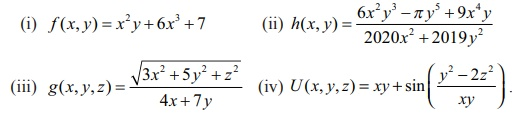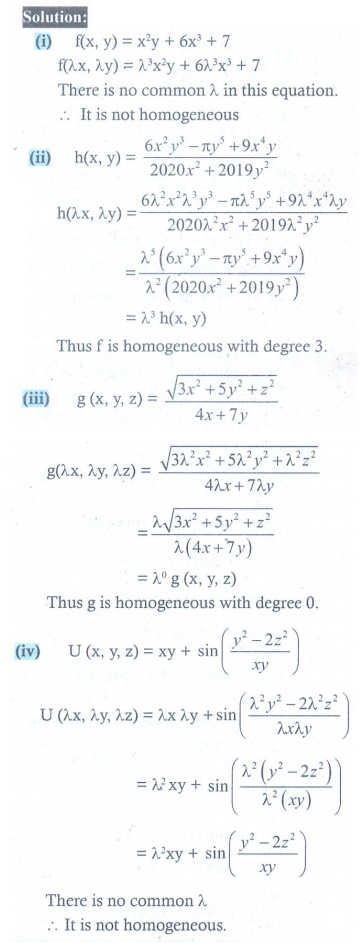Home | | Maths 12th Std | Exercise 8.7: Homogeneous Functions and Eulerãs Theorem

# Exercise 8.7: Homogeneous Functions and Eulerãs Theorem

Maths Book back answers and solution for Exercise questions - Mathematics : Differentials and Partial Derivatives: Homogeneous Functions and Eulerãs Theorem - Exercise Problem Questions with Answer, Solution

EXERCISE 8.7

1. In each of the following cases, determine whether the following function is homogeneous or not. If it is so, find the degree.2. Prove that f (xy= x3 ã 2x2y + 3xy2 + y3 is homogeneous; what is the degree? Verify Eulerãs Theorem for f .3. Prove that g(x, y) = x log (y/x) is homogeneous; what is the degree? Verify Eulerãs Theorem for g.